# The capacity of a passenger elevator is 2, 500 pounds. This load is supported by a...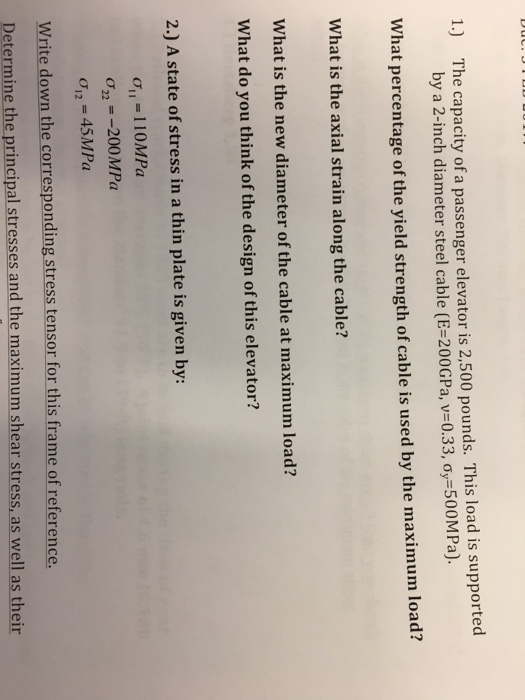The capacity of a passenger elevator is 2, 500 pounds. This load is supported by a 2-inch diameter steel cable (E=200GPa, v=0.33, sigma_y = 500MPa). What percentage of the yield strength of cable is used by the maximum load? What is the axial strain along the cable? What is the new diameter of the cable at maximum load? What do you think of the design of this elevator? A state of stress in a thin plate is given by: sigma_11 = 110MPa sigma_22 = 200MPa sigma_12 = 45MPa Write down the corresponding stress tensor for this frame of reference. Determine the principal stresses and the maximum shear stress, as well as their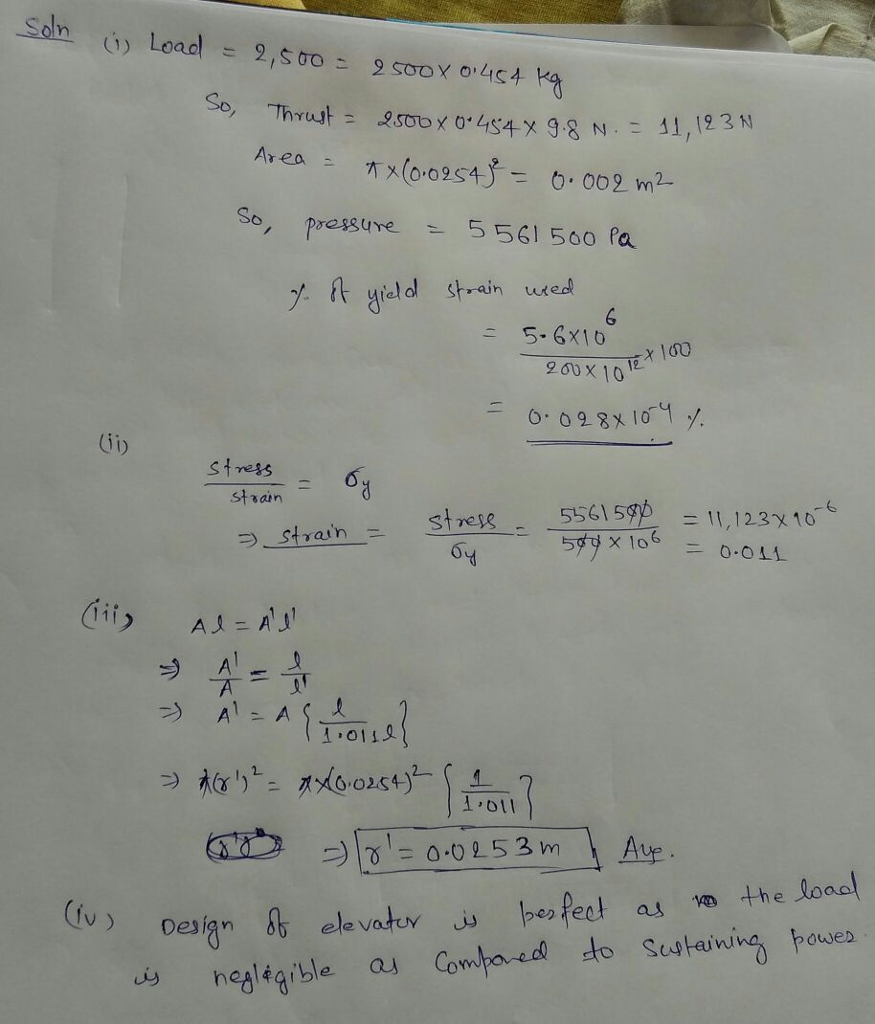#### Earn Coin

Coins can be redeemed for fabulous gifts.

Similar Homework Help Questions
• ### The shaft in the figure below is supported on journal bearings that do not offer any resistance t...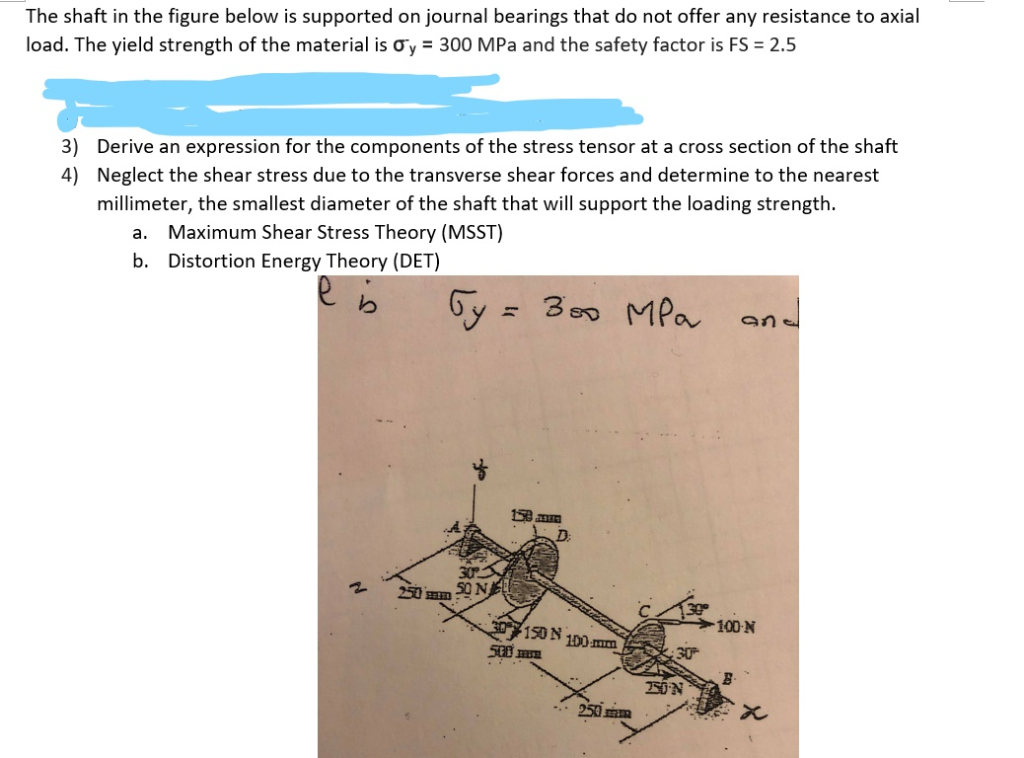The shaft in the figure below is supported on journal bearings that do not offer any resistance to axial load. The yield strength of the material is o",-300 MPa and the safety factor is FS-2.5 Derive an expression for the components of the stress tensor at a cross section of the shaft Neglect the shear stress due to the transverse shear forces and determine to the nearest millimeter, the smallest diameter of the shaft that will support the loading strength....

• ### The shaft in the figure below is supported on journal bearings that do not offer any resistance t...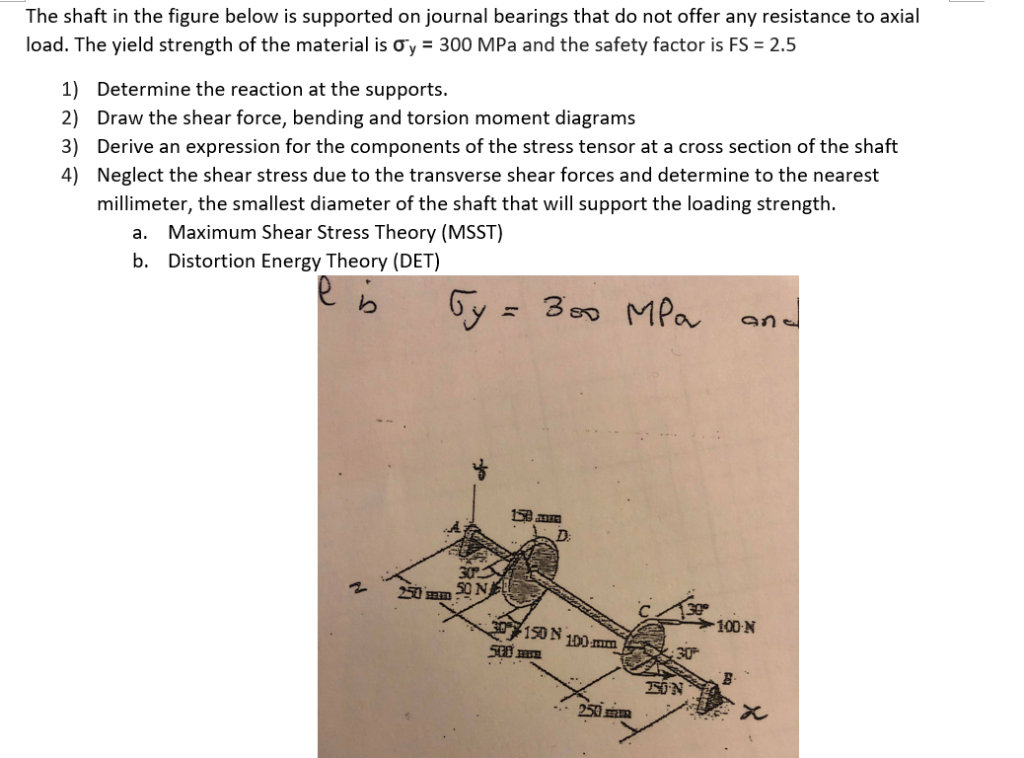The shaft in the figure below is supported on journal bearings that do not offer any resistance to axial load. The yield strength of the material is Ơ,-300 MPa and the safety factor is FS-2.5 1) 2) 3) 4) Determine the reaction at the supports. Draw the shear force, bending and torsion moment diagrams Derive an expression for the components of the stress tensor at a cross section of the shaft Neglect the shear stress due to the transverse shear...

• ### A simply supported prismatic beam is loaded with a load applied at an angle at point...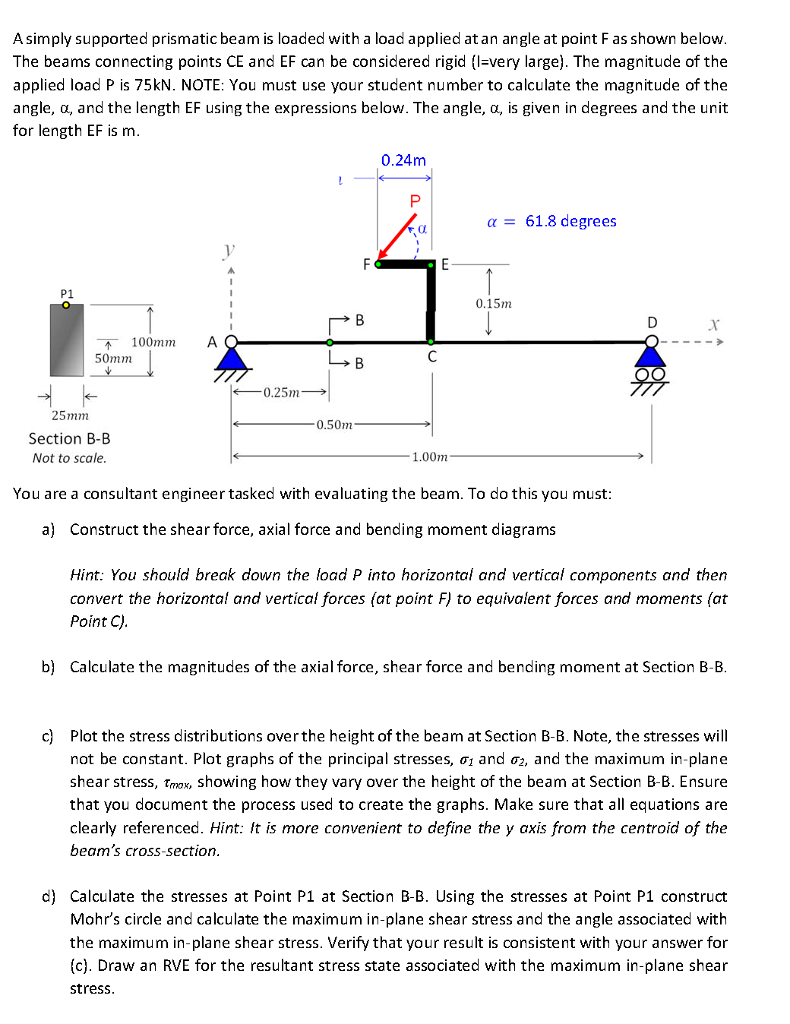A simply supported prismatic beam is loaded with a load applied at an angle at point F as shown below The beams connecting points CE and EF can be considered rigid (l-very large). The magnitude of the applied load P is 75kN. NOTE: You must use your student number to calculate the magnitude of the angle, α, and the length EF using the expressions below. The angle, α, is given in degrees and the unit for length EF is m...

• ### 5) Load Combination and Mohr Circle: The beveled 2 gear is subjected to the loads shown....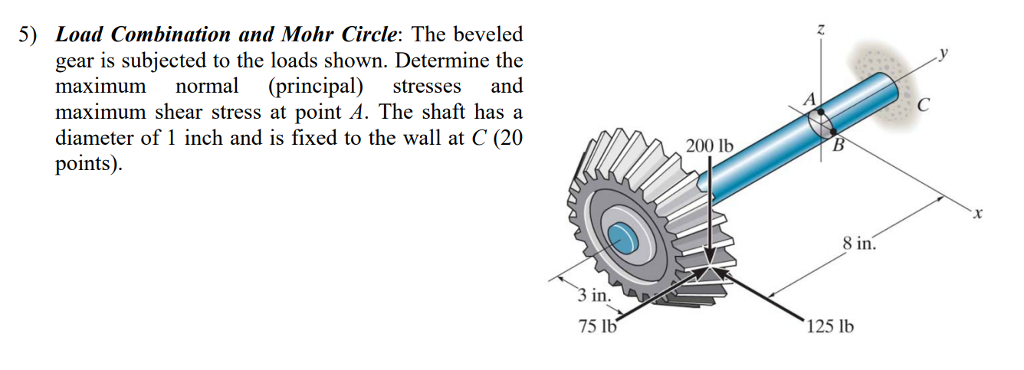5) Load Combination and Mohr Circle: The beveled 2 gear is subjected to the loads shown. Determine the maximum normal (principal) stresses and maximum shear stress at point A. The shaft has a diameter of 1 inch and is fixed to the wall at C (20 points) 200 lb 8 in. in. 75 lb 125 lb

• ### Below is a diagram of a beam that is simply supported and subjected to a point load over a 9.8 in...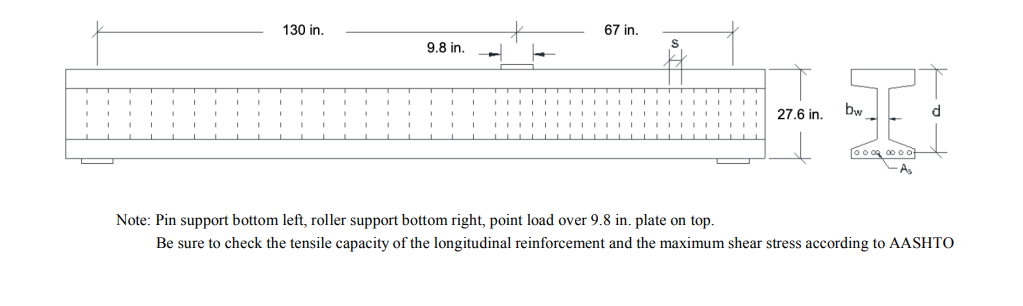Below is a diagram of a beam that is simply supported and subjected to a point load over a 9.8 in. plate. The member has 6.14 sq.-in. of flexural tension steel. You can use d = 25.7 in., bw = bv = 3.54 in., f’c = 12.2 ksi, fy = 87 ksi, Av = 0.157 sq.-in. at a spacing s = 6.50 in., finally, the maximum course aggregate used in the beams was 0.50 in. There is no axial load...

• ### Questions 5-8 A steel frame shown below is subjected to combined uniformly distributed gravity load (w...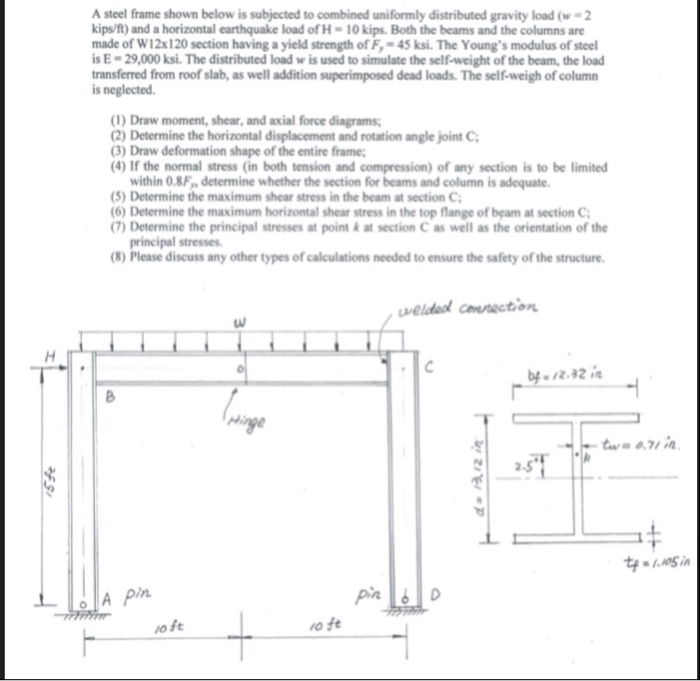Questions 5-8 A steel frame shown below is subjected to combined uniformly distributed gravity load (w 2 kips/f) and a horizontal earthquake load of H-10 kips Both the beams and the columns are made of W12x120 section having a yield strength of F 45 ksi. The Young's modulus of steel s E-29,000 ksi. The distributed load w is used to simulate the self-weight of the beam, the load transferred from roof slab, as well addition superimposed dead loads. The self-weigh...

• ### A 3 m rigid bar AB is supported with a vertical translational spring at A and a pin at B The bar is subjected to a linearly varying distributed load with maximum intensity g Calculate the ver...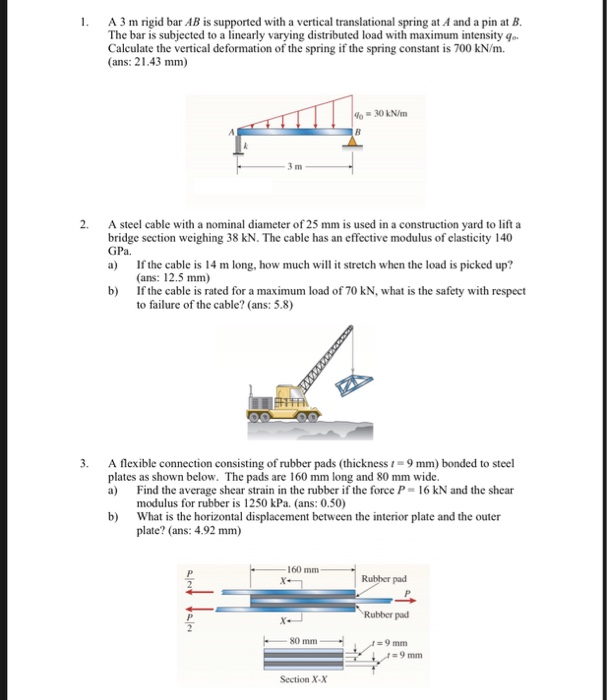A 3 m rigid bar AB is supported with a vertical translational spring at A and a pin at B The bar is subjected to a linearly varying distributed load with maximum intensity g Calculate the vertical deformation of the spring if the spring constant is 700 kN/m. (ans: 21.43 mm) 2. A steel cable with a nominal diameter of 25 mm is used in a construction yard to lift a bridge section weighing 38 kN. The cable has an...

• ### A shaft subjected to bending moment varying from -200 N m to +500 N m and a varying torque from 50 N m to 175 N m. If material of the shaft is 30C8, stress concentration factor is 1.85, notch sensitivity is 0.95 reliability 99.9% and factor of safety is 1

A shaft subjected to bending moment varying from -200 N m to +500 N m and a varying torque from 50 N m to 175 N m. If material of the shaft is 30C8, stress concentration factor is 1.85, notch sensitivity is 0.95 reliability 99.9% and factor of safety is 1.5, find the diameter of the shaft. The load on a bolt consists of an axial pull of 10 kN together with a transverse shear force of 5 kN. Find...

• ### 4.A thin-walled cylindrical column carries a weightless arch and is loaded as shown in Figure 4. The column has an internal diameter of 250 mm with a thickness of 2 mm Given: E-200 GPa and 0.3 The an...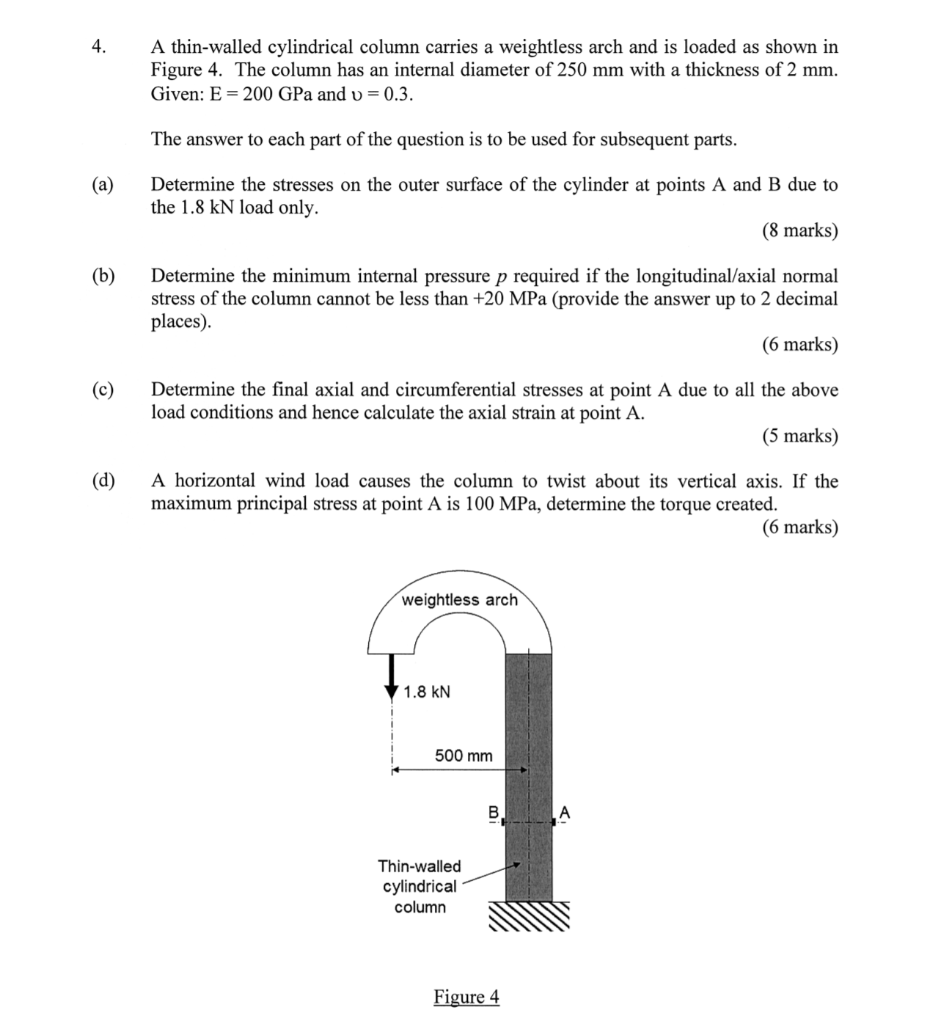4.A thin-walled cylindrical column carries a weightless arch and is loaded as shown in Figure 4. The column has an internal diameter of 250 mm with a thickness of 2 mm Given: E-200 GPa and 0.3 The answer to each part of the question is to be used for subsequent parts (a)Determine the stresses on the outer surface of the cylinder at points A and B due to (8 marks) (b) Determine the minimum internal pressure p required if the...

• ### scenes M4.3 Load capacity of beam-strut structure The structure supports a distributed load of w. The...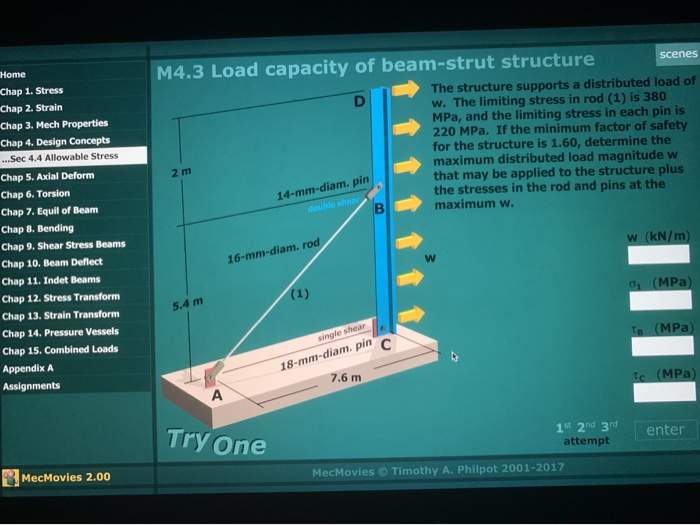scenes M4.3 Load capacity of beam-strut structure The structure supports a distributed load of w. The limiting stress in rod (1) is 380 MPa, and the limiting stress in each pin is 220 MPa. If the minimum factor of safety for the structure is 1.60, determine the 2 m maximum distributed load magnitude w that may be applied to the structure plus the stresses in the rod and pins at the maximum w. 14-mm-diam. pin Home Chap 1. Stress Chap...

Free Homework Help App